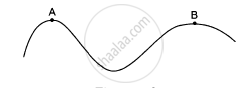Share

# A Car Moves at a Constant Speed on a Road as Shown in Figure. the Normal Force by the Road on the Car Na and Nb When It is at the Points a and B Respectively. - Physics

ConceptCircular Motion

#### Question

A car moves at a constant speed on a road as shown in figure. The normal force by the road on the car NA and NB when it is at the points A and B respectively.• NA = NB

• NA > N

• NA < N

•  insufficient

#### Solution

NA < NB
From the figure in the question, it is clear that $r_B > r_A$.

Here, normal reaction is inversely proportional to the centrifugal force acting on the car, while taking turn on the curve track. Also, centrifugal force is inversely proportional to the radius of the circular track.
Therefore, we have:
NA < N

Is there an error in this question or solution?

#### APPEARS IN

Solution A Car Moves at a Constant Speed on a Road as Shown in Figure. the Normal Force by the Road on the Car Na and Nb When It is at the Points a and B Respectively. Concept: Circular Motion.
S